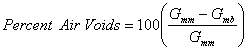# Compaction Measurement and Reporting

Compaction reduces the volume of air in HMA. Therefore, the characteristic of concern is the volume of air within the compacted pavement. This volume is typically quantified as a percentage of air voids by volume and expressed as “percent air voids”. Percent air voids is calculated by comparing a test specimen’s bulk density with its theoretical maximum density (TMD) and assuming the difference is due to air. Once TMD is known, portable devices can be used to measure HMA density in-place. The terms “percent air voids” and “density” are often used interchangeably. Although this is not wrong, since density is used to calculate percent air voids, the fundamental parameter of concern is always percent air voids.

Percent air voids is typically calculated by using AASHTO T 269, ASTM D 3203 or an equivalent procedure (AASHTO, 2000). These procedures all use laboratory-determined bulk specific gravity and theoretical maximum specific gravity in the following equation:where: Gmm = theoretical maximum specific gravity of the particular HMA in question Gmb = bulk specific gravity of the HMA in question

These procedures require a small pavement core (usually 100 – 150 mm (4 – 6 inches) in diameter), which is extracted from the compacted HMA (see Figure 1 and 2). This type of air voids testing is generally considered the most accurate but is also the most time consuming and expensive.

Since core extraction is time consuming and expensive, air voids are often measured indirectly using a portable density-measuring device such as a nuclear density gauge (see Figure 3) or electrical density gauge (see Figure 4).